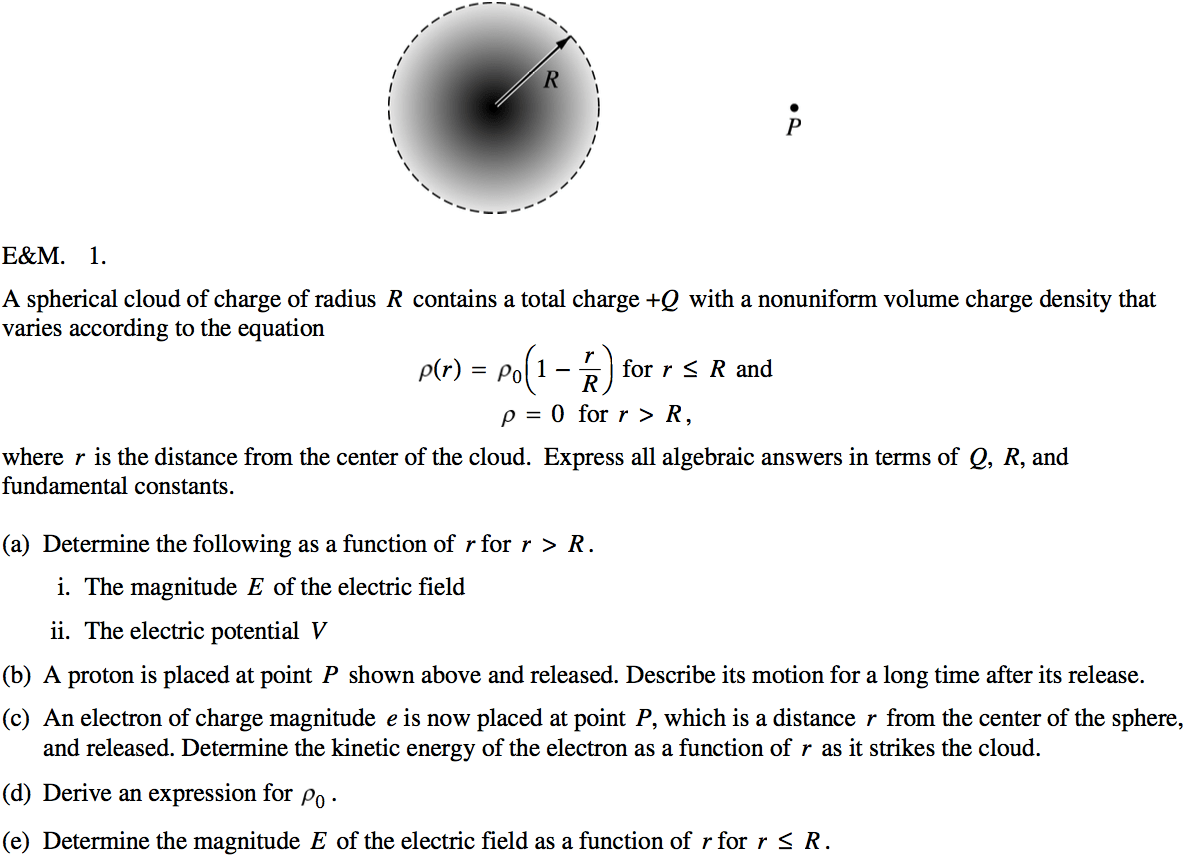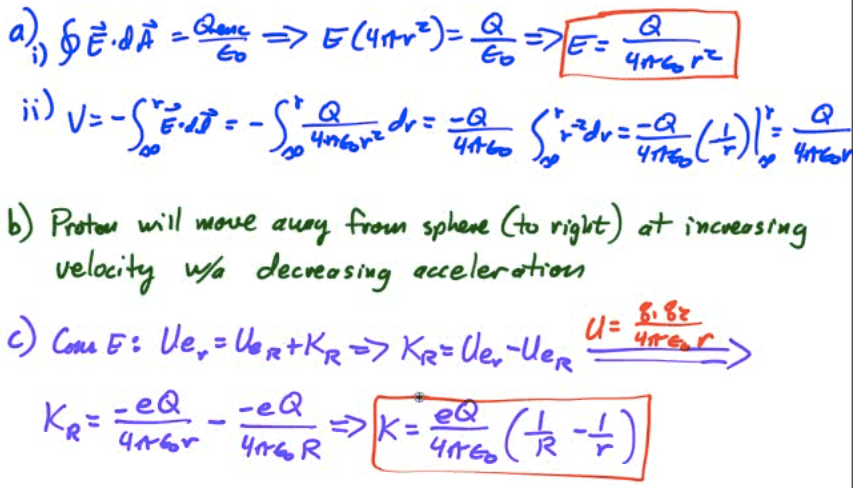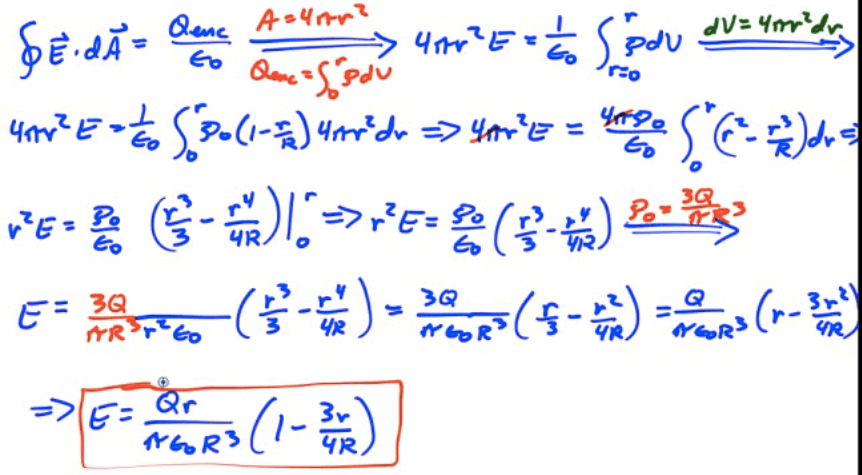# Electric Potential Energy

• When an object was lifted against gravity by applying a force for some distance, work was done to give that object gravitational potential energy

• When a charged object is moved against an electric field by applying a force for some distance, work is done to give that object electric potential energy.

• The work done per unit charge in moving a charge between two points in an electric field is a scalar known as the electric potential V (or voltage)

• Units: volts (1V = 1 J/C)

• The work done is equal to the change in the object's electric potential energy (UE)

•# The Electron-Volt

• Oftentimes the electrical energy and/or work done is a very small portion of a joule

• A smaller, alternative, non-standard unit of energy is often used for convenience, known as the electron volt (eV)

• 1 eV is the amount of work done in moving an elementary charge through a potential difference of 1 volt

•# Equipotential Lines

• Topographic maps show you lines of equal altitude, or equal gravitational potential

• Lines connecting points of equal electrical potential are known as equipotential lines.

• Equipotential lines always cross electrical field lines at right angles

• If you move a charged particle in space, and stay on an equipotential line, no work will be done.

• As equipotential lines get closer together, the gradients of the potential increases (stepper "slope" of potentials)

• Electric field points from high to low potential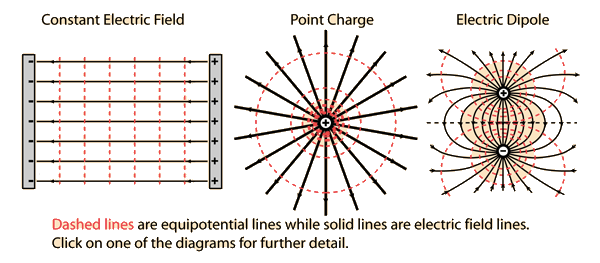# Electric Potential Energy Due to a Point Charge

••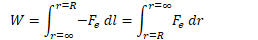••# Electric Force from Electric Potential Energy

•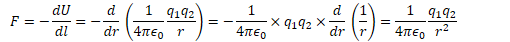# Electric Potential Due to a Point Charge

• Electric potential (voltage) is the work per unit charge required to bring a charge from infinity to some point R in an electric field

•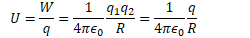•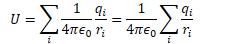# Example 1: Electric Potential Due to a Point Charge

• Find the electric potential at point P, located 3 meters away from a -2 C charge.

• What is the electric potential energy of a 0.5 C charge situated at point P?# Example 2: Electric Potential Due to a Point Charge

• Find the electric potential at the origin due to the three charges shown in the diagram. If an electron is placed at the origin, what potential energy does it possess?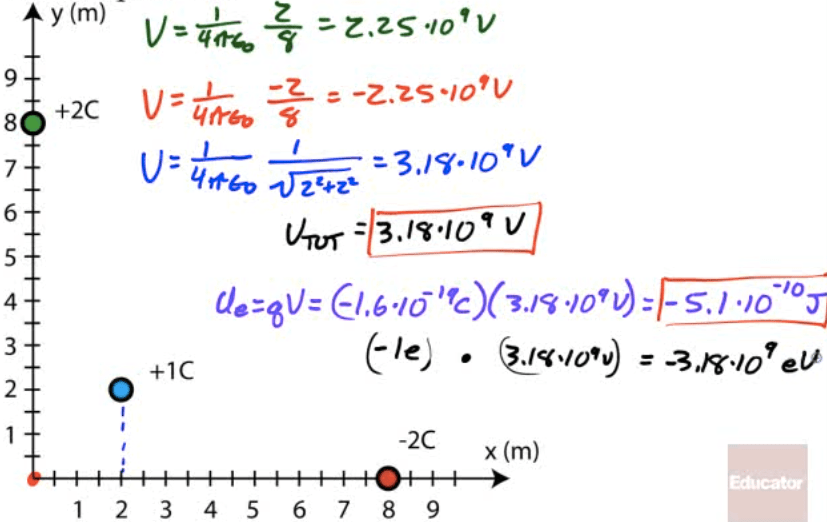# Finding Electric Field from Electric Potential

•••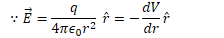# Finding Electric Potential from Electric Field

•••# Example 3: Electric Potential Due to a Point Charges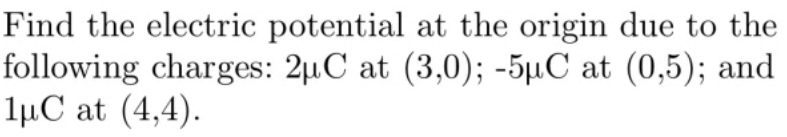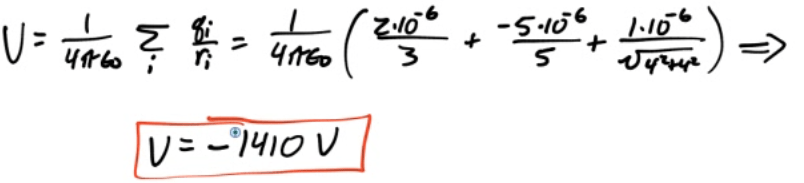# Example 4: Electric Field from Potential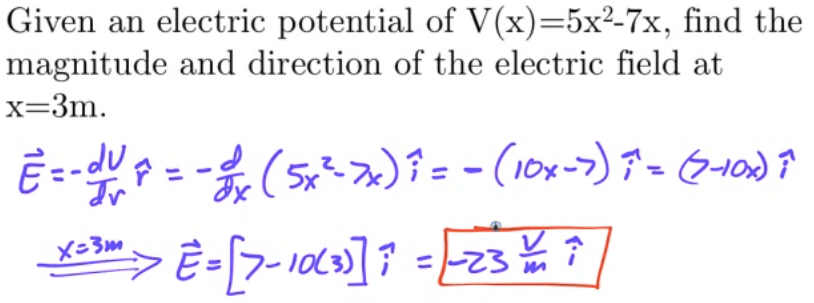# Example 5: Speed of an Electron Released in an Electric Field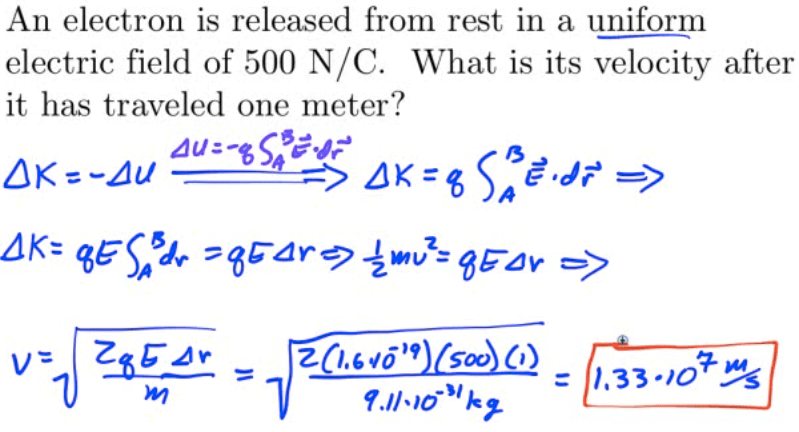# Example 6: Work Required to Establish a Charge System

![Two point charges (5 VLC and 2 [LC) are placed 0.5 meters apart. How much work was required to establish the charge system? ](./media/image103.png)# 2013 Free Response Question 1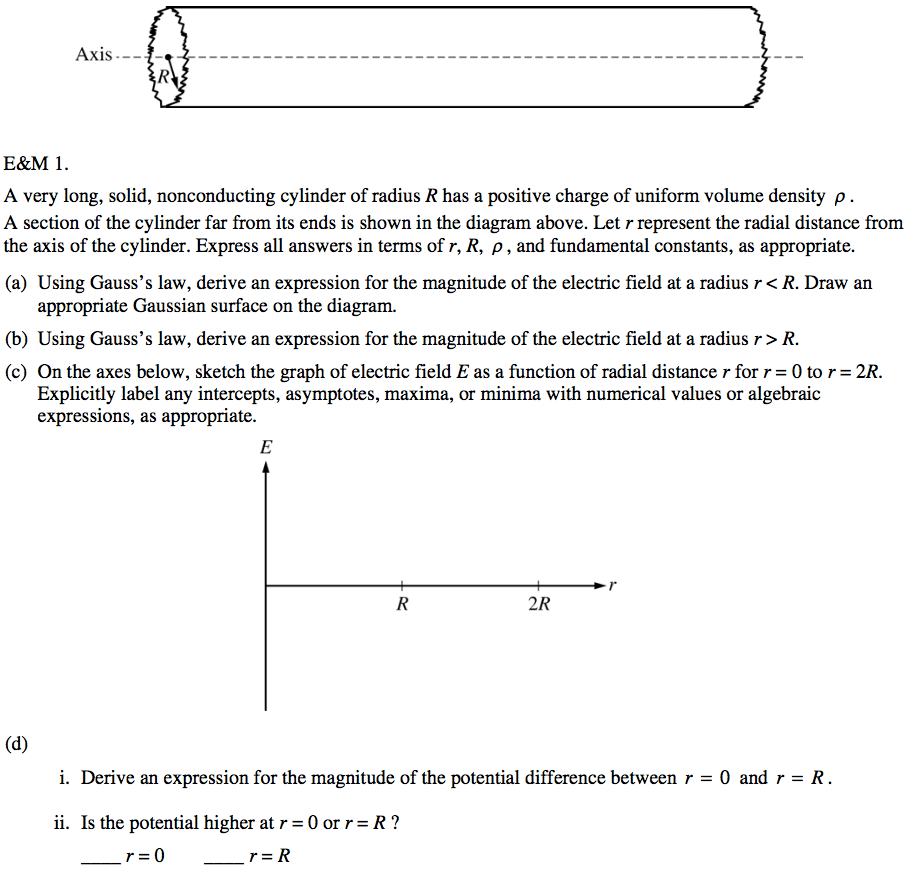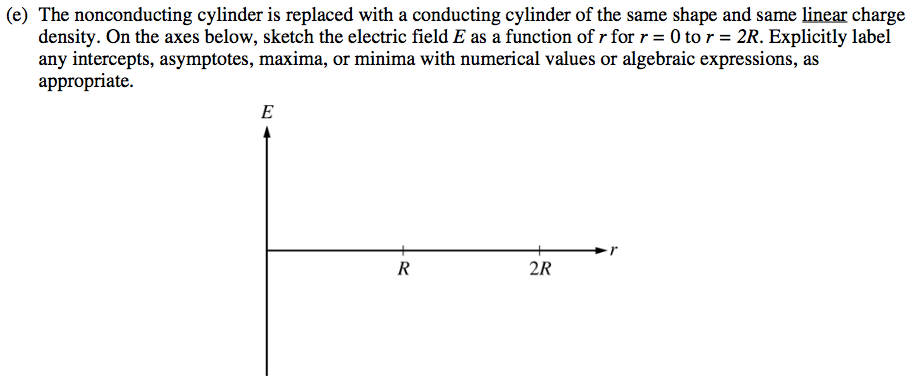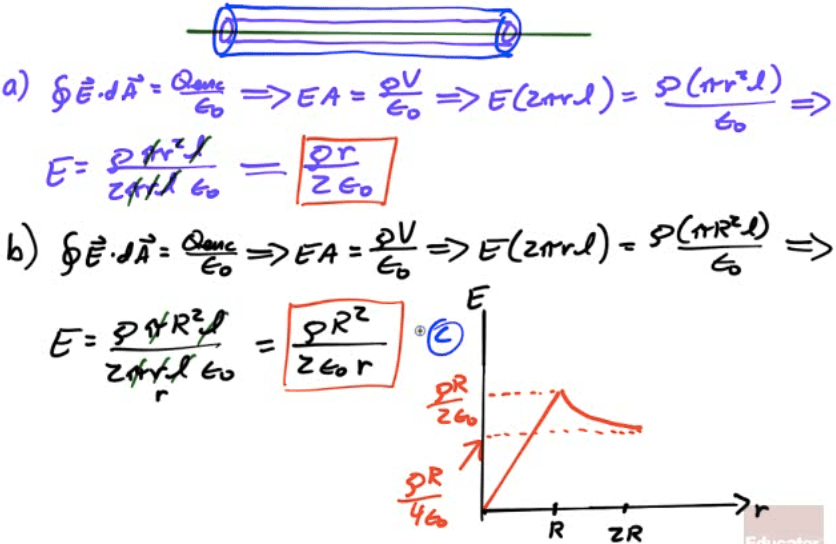![[9-/nl 2-4 ](./media/image108.png)# 2006 Free Response Question 1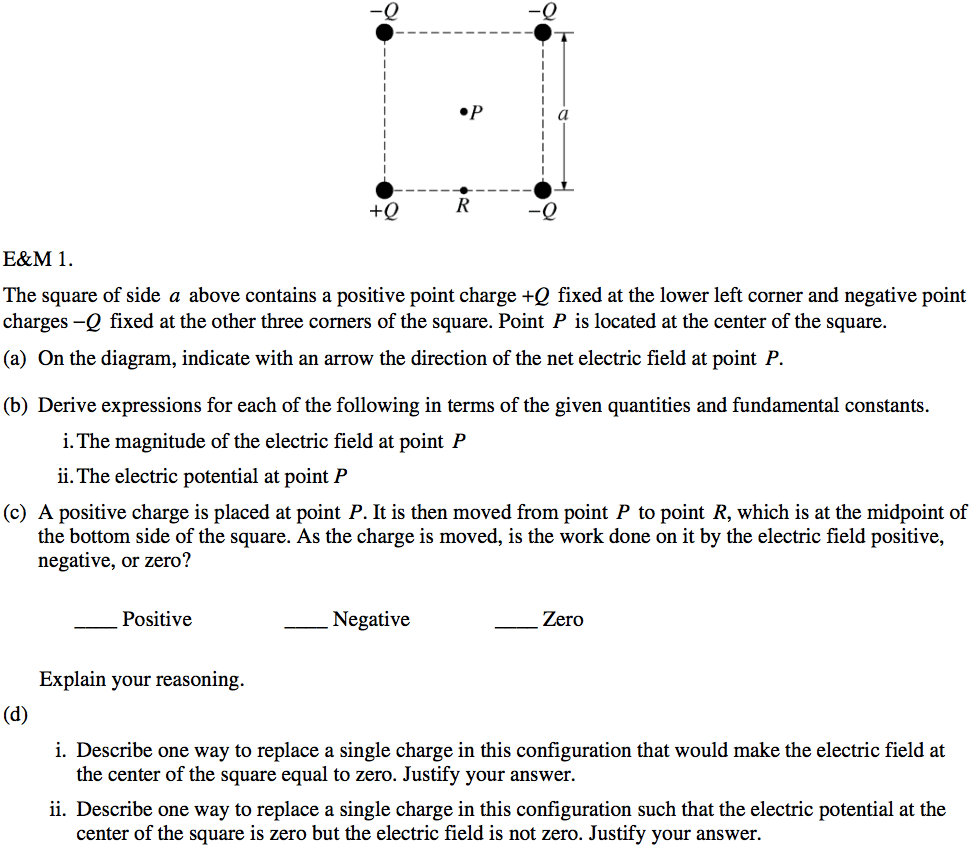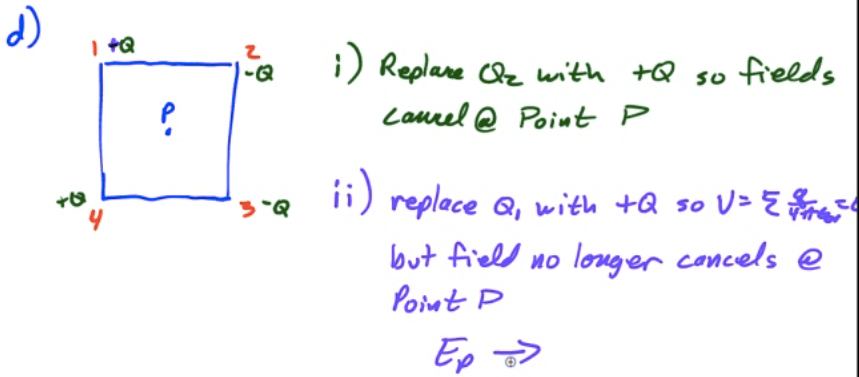# 2005 Free Response Question 1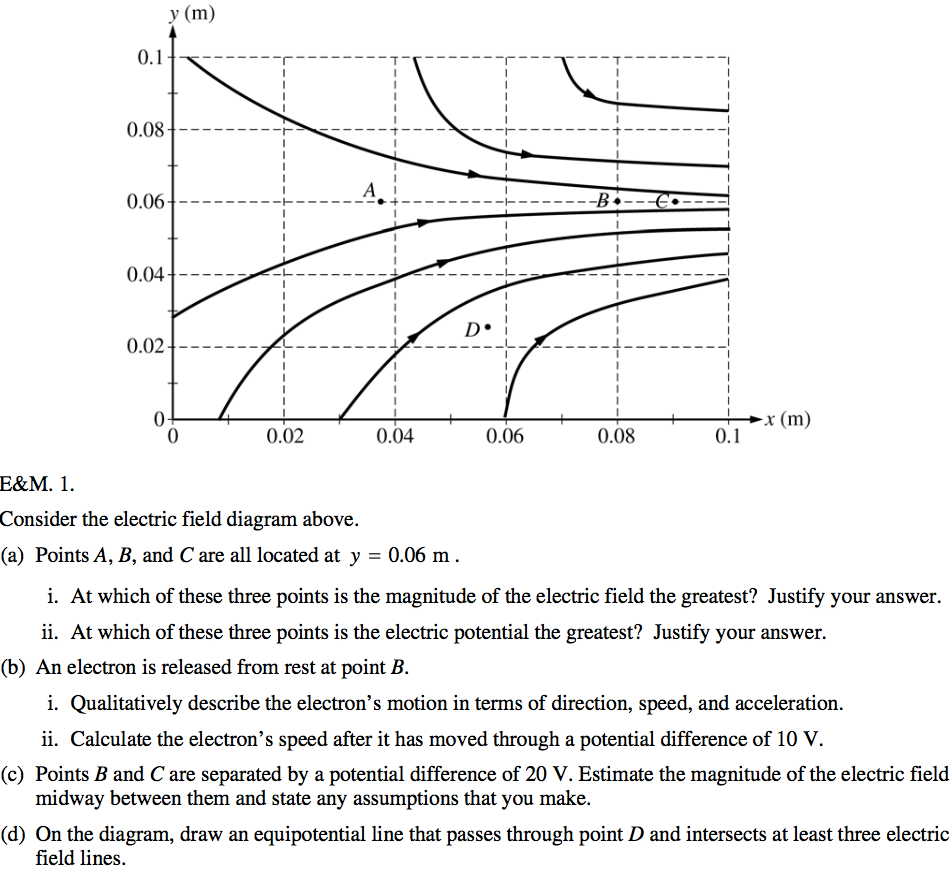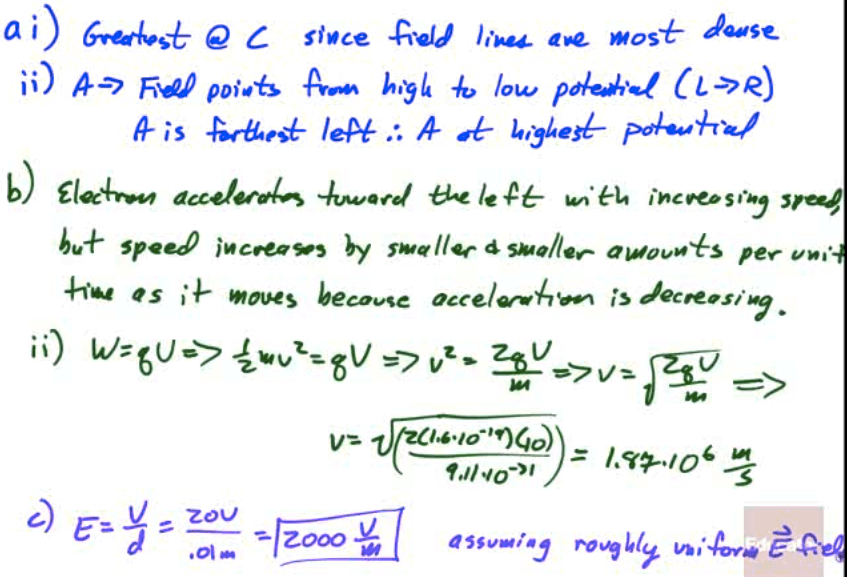# 2003 Free Response Question 1